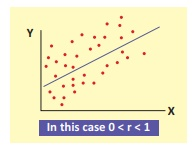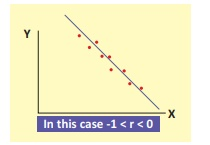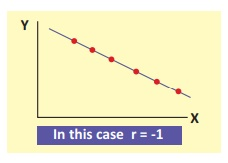Home | | Statistics 12th Std | Scatter Diagram

# Scatter Diagram

A scatter diagram is the simplest way of the diagrammatic representation of bivariate data. One variable is represented along the X-axis and the other variable is represented along the Y-axis.

SCATTER DIAGRAM

A scatter diagram is the simplest way of the diagrammatic representation of bivariate data. One variable is represented along the X-axis and the other variable is represented along the Y-axis. The pair of points are plotted on the two dimensional graph. The diagram of points so obtained is known as scatter diagram. The direction of flow of points shows the type of correlation that exists between the two given variables.

### 1) Positive correlation

If the plotted points in the plane form a band and they show the rising trend from the lower left hand corner to the upper right hand corner, the two variables are positively correlated.### 2) Negative correlation

If the plotted points in the plane form a band and they show the falling trend from the upper left hand corner to the lower right hand corner, the two variables are negatively correlated.### 3) Uncorrelated

If the plotted points spread over in the plane then the two variables are uncorrelated.### 4) Perfect positive correlation

If all the plotted points lie on a straight line from lower left hand corner to the upper right hand corner then the two variables have perfect positive correlation.### 5) Perfect Negative correlation

If all the plotted points lie on a straight line falling from upper left hand corner to lower right hand corner, the two variables have perfect negative correlation.## Merits and Demerits of scatter diagram

### Merits

Â·              It is a simple and non-mathematical method of studying correlation between the variables.

Â·              It is not influenced by the extreme items

Â·              It is the first step in investigating the relationship between two variables.

Â·              It gives a rough idea at a glance whether there is a positive correlation, negative correlation or uncorrelated.

### Demerits

Â·              We get an idea about the direction of correlation but we cannot establish the exact strength of correlation between the variables.

Â·              No mathematical formula is involved.

Tags : Merits and Demerits | Correlation Analysis , 12th Statistics : Chapter 4 : Correlation Analysis
Study Material, Lecturing Notes, Assignment, Reference, Wiki description explanation, brief detail
12th Statistics : Chapter 4 : Correlation Analysis : Scatter Diagram | Merits and Demerits | Correlation Analysis